Next: 4.2.3 Method Up: 4.2 Formalism Previous: 4.2.1 Model

## 4.2.2 Electron-Phonon Coupling

In this chapter, we define the dimensionless electron-phonon coupling as the ratio of the lattice deformation energy of a localized electron and the kinetic (delocalization) energy of the electron. This definition is encountered mostly in polaron studies focusing on the transition from large to small polarons (30,28). For the Holstein model the deformation energy is given by (see Eq. 4.37)(4.6)

and the dimensionless coupling is defined as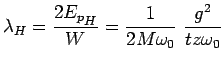(4.7)

where the half-bandwidth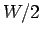is taken as a measure of the free electron kinetic energy. For a simple tight binding dispersion, whereis the coordination number.

Analogously, for the breathing model the lattice deformation energy is (see Appendix 4.6.2)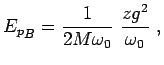(4.8)

and the dimensionless coupling is hence defined as(4.9)

Compared to the Holstein case, the lattice deformation energy for the breathing model has an extra factor ofas can be seen in Eq. 4.8, which results form the fact that the electron interacts withneighboring oscillators.

There are several other definitions for the dimensionless electron-phonon coupling throughout the literature. For instance in the BCS theory the definition of the dimensionless coupling is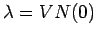, whereand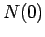are the effective electron-electron attraction and respectively the density of states at the Fermi level. In the weak coupling regime the effective interaction at small frequency or in the antiadiabatic limit (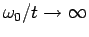) is(4.10)

If the integration over allmomenta is considered for the breathing model, then(4.11)(4.12)

When choosing the density of states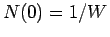, this definition of the dimensionless coupling coincides with our definition.

In the Migdal-Eliashberg theory of superconductivity,where only scattering processes at the Fermi surface are considered (31,32) in. This definition is equivalent to(32)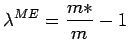(4.13)

whereis the quasiparticle renormalized effective mass at the Fermi surface.can be directly determined from experiments sinceand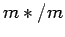can be measured in tunneling (33), neutron (34) or respectively in the ARPES experiments (2). In our case, the effective mass at the bottom of the band (which is the zero energy state of our system and in many respects similar to the Fermi surface in a many-electron system) can be determined from the equation(4.14)

valid at small. The ratio of the effective mass and the un-renormalized mass is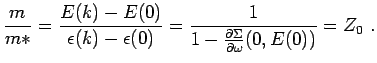(4.15)

Therefore, we have(4.16)

This definition directly relates the quasiparticle weight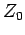to the coupling constant. In the first order perturbation theory one gets(4.17)

By inspecting Eq. 4.17 one can see that the most important contribution to the integral comes form smallwhere the denominator is small. For the B model this will introduce a significant difference betweenand our(Eq. 4.9). Unlike our definition which assumes average over all possible phonon momenta,is determined by smallphonon scatterings which are characterized by small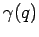in the B model.

The definition ofused in the Migdal-Eliashberg theory was intended to describe the properties at the Fermi level or at small energy excitation. It does not properly describe the physics at larger energy since the most relevant scattering processes in this case imply larger phonon momenta as we will discuss in the next two sections.Next: 4.2.3 Method Up: 4.2 Formalism Previous: 4.2.1 Model
© Cyrill Slezak